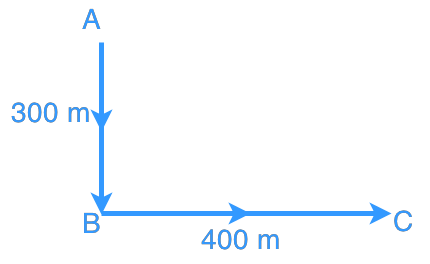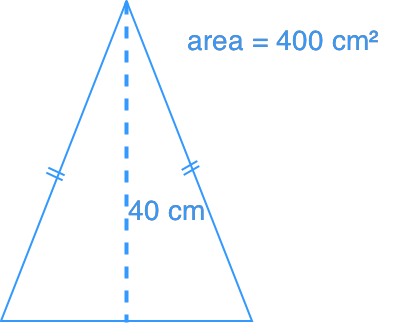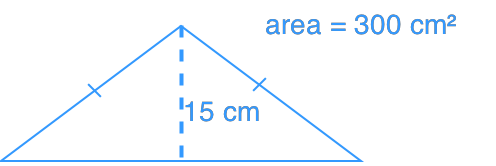Applications of pythagorean theorem

Applications of pythagorean theorem

We will need to apply Pythagorean Theorem often in our daily life. In this lesson, we will focus on tackling some Pythagorean Theorem word problems.

Lessons

• Introduction
a)

i) What is Pythagorean Theorem?

ii) How to use Pythagorean Theorem to find sides of a triangle?

b)
What are Pythagorean Triples?

• 1.
An aeroplane flew from point A to point B, and then to Point C. How far is the plane from point A? Refer to the diagram below.• 2.
A 2.5 m long ladder is put against a wall. If the base of the ladder is 1.25 m away from the wall, what is the length from the ground to the point where the top of ladder touches the wall?

• 3.
Which triangle has a longer perimeter?
Triangle ATriangle B